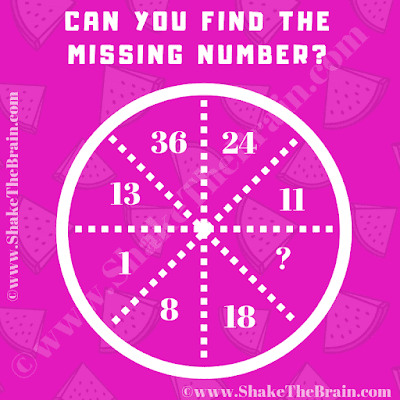This is a mind-challenging missing number maths circle puzzle which will test your IQ. In this missing number puzzle, you are shown some numbers inside a Circle. Your challenge is logical or mathematical relate these given numbers and then find the value of the missing number which will replace the question mark.Can you find the value of the missing number?
The answer to this "Missing Number Maths Circle Puzzle to Test your IQ", can be viewed by clicking on the answer button.